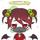## Social Question# Can you find the trick to solving this math problem?

Asked by LostInParadise (29638) June 24th, 2020

I thought of this math problem, though I am certainly not the first to think of it. It has a rather simple solution if you think about it the right way.

What is the most numbers that you can choose from 1 to 100 such that no number is twice as great as any other number?

The most natural way to attack this is to choose numbers starting at 1 and skipping over numbers that are twice as great as numbers previously chosen. There is a much slicker way. Start choosing numbers beginning at 100 and move downward. Do you see the trick?

Observing members: 0Composing members: 0Would that not be every uneven number?

ragingloli (49928)“Great Answer” (3) Flag as…You can do better. For example, you could choose all the uneven numbers and also include 4, 4×3, 4×5, 4×7,...,4×25. In fact, this leads to another solution. Do you see how to complete what I started? There are four more numbers that can be added.

LostInParadise (29638)“Great Answer” (1) Flag as…Perhaps I should have stated the problem more clearly. What I mean was that no number among those chosen is twice as great as any of the other numbers chosen.

LostInParadise (29638)“Great Answer” (1) Flag as…I see I have no takers. This is the approach that I had in mind. We want to choose as many numbers as possible from 1 to 100 without any of them being twice as large as any other.

Start by choosing 100. We can also choose 99 and 98 and, hold on. We can choose all the numbers from 51 to 100. Obviously none is twice as large as any other.

Continuing, we can’t choose 50, since 100 is twice 50, we can’t choose 49, and we can’t choose any number down to 26.

So we have – include 51 to 100, exclude 26 to 50. Here is the fun part. We can now choose 13 to 25, because twice these numbers go from 26 to 50, which were excluded.

Continuing – exclude 7 to 12, include 4 to 6, exclude 2 to 3, include 1.
Summing up, we get 1, 4, 5, 6, 13 to 25, and 51 to 100, giving us 4 + 13 + 50 = 67 of the numbers from 1 to 100.

The approach started by @ragingloli also works, but may be a little more difficult to undersand.. We use the fact that any number can be expressed as (2**k)x where x is odd. (See if you can convince yourself that this is true.) If we choose only even values of k, no number will be twice as large as any other. Start by including all the odd numbers, corresponding to k=0. We don’t want twice any of these numbers, but we can include 4 times the odd numbers (corresponding to k=2) giving 4x for odd values of x from 1 to 25. We can’t include 8 times the odd numbers, but we can include 16 (16×1 and 16×3 and 16×5) and 64 (64×1) times the odd numbers. We end up with:
All odd numbers
4x for odd values x from 1 to 25
16, 48 and 80
64

This gives 50 + 13 + 3 + 1 = 67 values. The same number as before, but with different values.

LostInParadise (29638)“Great Answer” (1) Flag as…